# How to Subtotal for Value in Specific Cell in Excel

Sometimes we may want to sum within some criteria in excel, actually, we can use SUMIF function directly instead of SUM for some special instance. For example, if we just want to get subtotal for one product among all products, we can use SUMIF function here to only calculate the total for the specific product properly. In this tutorial, we will show you how to subtotal for the value in a specific cell in worksheet.

Precondition:

Prepare tables in a worksheet like below: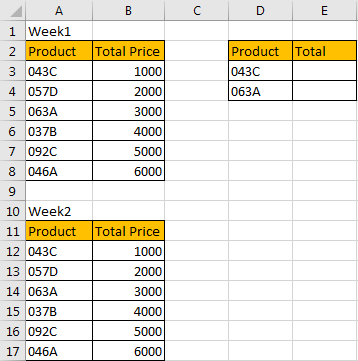We can use SUM function to calculate total price for all products in a week. But If we only want to subtotal for product ‘043C’ or ‘063A’ during two weeks, how can we do?

Method: Subtotal for Value in Specific Cell by Formula

Step 1: In E2 which is just net to product ‘043C’, enter the formula:

=SUMIF(\$A\$3:\$A\$17,D3,\$B\$3:\$B\$17).

In this formula, \$A\$3:\$A\$17 this selected range will provide all criteria, we add \$ before row and column to make this range as an absolute reference, so if you want to copy down this formula, the range will not be changed. D3 is the sum criteria in the formula, if D3 can be found in \$A\$3:\$A\$17, then mapping value from \$B\$3:\$B\$17 will be summed for D3.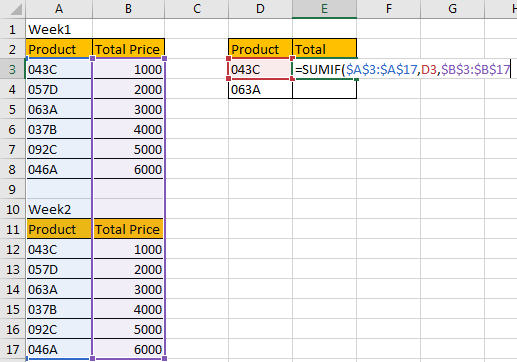Step 2: Click Enter to get result. Verify that correct value 2000 is returned.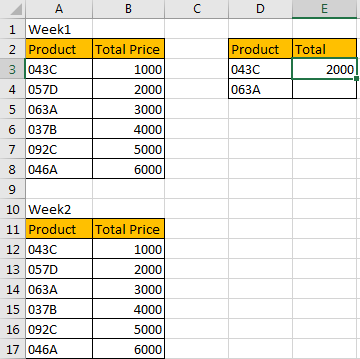Step 3: Drag the handle down to copy the formula. Verify that correct value 6000 is returned.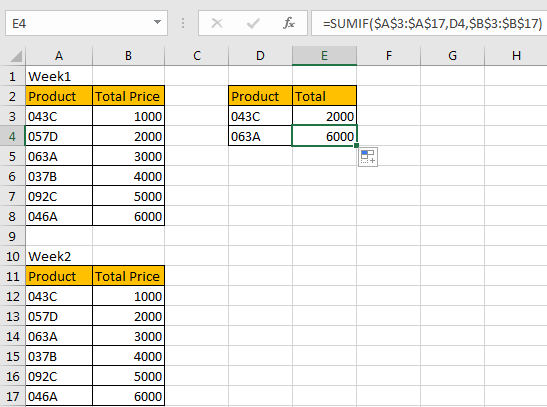Step 4: If you change product in D3, the subtotal value is updated accordingly. For example, copy ‘037B’ to D3, then subtotal is recalculated and updated immediately. So, you can copy product to this cell then you can get subtotal for this product, D3 is the specific cell to show the criteria.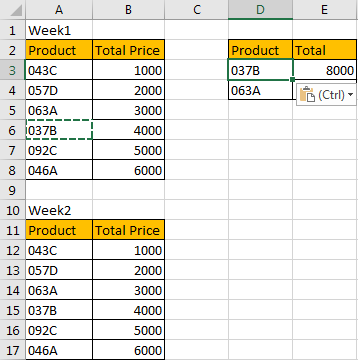Note:

In this instance, if the range is long, you can also use formula =SUMIF(A:A,D3,B:B) to get subtotal for specific cell.

### Related Functions

• Excel SUMIF Function
The Excel SUMIF function sum the numbers in the range of cells that meet a single criteria that you specify. The syntax of the SUMIF function is as below:=SUMIF (range, criteria, [sum_range])…